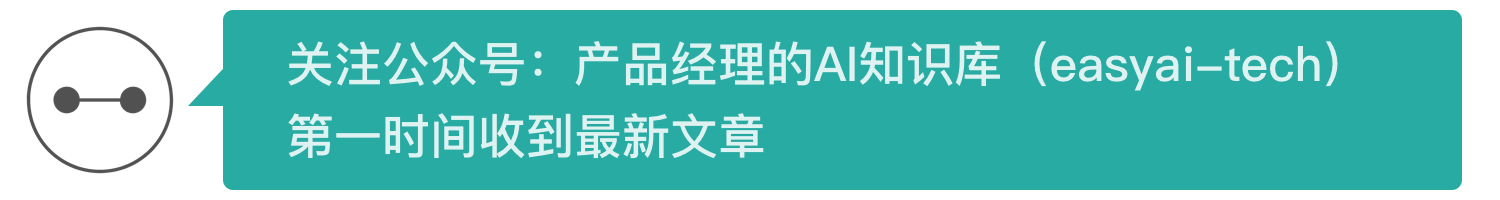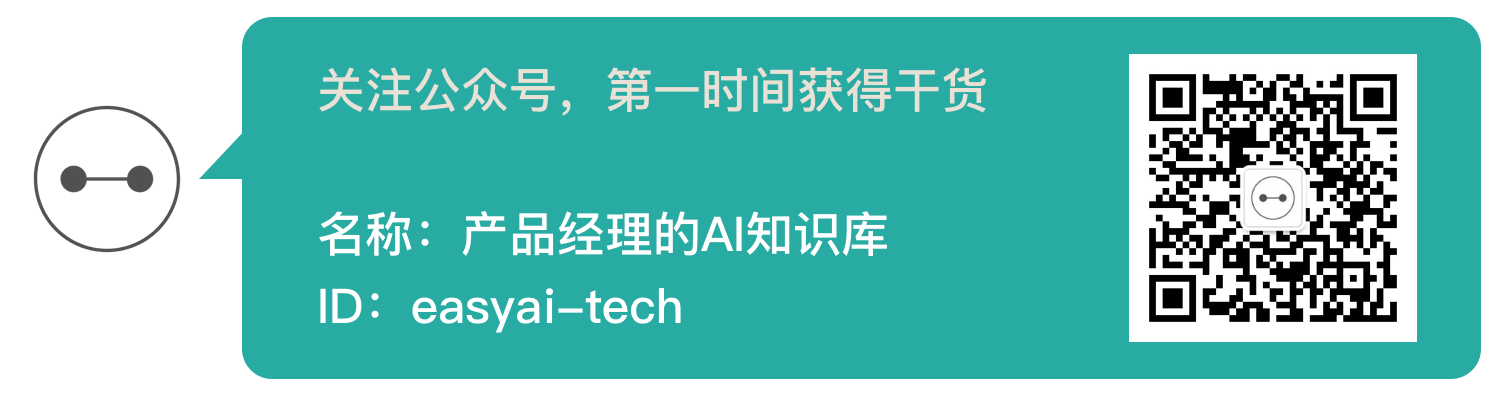【新智元导读】Unsupervised learning is a type of machine learning technique used to discover patterns in data. This paper introduces several clustering algorithms for unsupervised learning in Python, including K-Means clustering, hierarchical clustering, t-SNE clustering, and DBSCAN clustering.

Unsupervised learning is a type of machine learning technique used to discover patterns in data. The data of the unsupervised algorithm is not labeled, which means that only the input variable (X) is provided and there is no corresponding output variable. In unsupervised learning, the algorithm itself discovers meaningful structures in the data.

Yan Lecun, Facebook's chief AI scientist, explains that unsupervised learning—that is, teaching machines to learn on their own—does not need to explicitly tell whether everything they do is right or wrong, and is the key to “real” AI.

## Supervised learning vs unsupervised learning

In supervised learning, the system attempts to learn from the examples given earlier. Conversely, in unsupervised learning, the system attempts to find patterns directly from the given examples. Therefore, if the dataset has a tag, it is a supervised issue, and if the dataset is unmarked, it is an unsupervised issue.

As shown above, the left side is an example of supervised learning; we use regression techniques to find the best fit line between features. In unsupervised learning, input is based on feature separation, and prediction depends on which cluster it belongs to.

Important term

• Feature: The input variable used to make the prediction.
• Predictions: The output of the model when an input example is provided.
• Example: A row of the data set. An example contains one or more features and may have tags.
• Label: The result of the feature.

## Preparing for unsupervised learning

In this article, we useIris dataset (Iris flower dataset)Come and make our first prediction. The data set contains a set of data for 150 records, with 5 attributes - petal length, petal width, sepal length, sepal width and category. The three categories are Iris Setosa (Imperial Shan), Iris Virginica (Virginia Iris) and Iris Versicolor (Discoloration Iris). For our unsupervised algorithm, we give these four characteristics of the iris and predict which category it belongs to. We use the sklearn Library in Python to load Iris data sets and use matplotlib for data visualization. Below is the code snippet.

## Clustering

In clustering, data is divided into groups. Simply put, the purpose is to open the components with similar characteristics and cluster them into groups.

Visualization example:In the above figure, the image on the left is the raw data of the unfinished classification, and the image on the right is clustered (the data is classified according to the characteristics of the data). When an input to be predicted is given, it is checked in its cluster and predicted based on its characteristics.

## K-Means clustering in Python

K-Means is a kindIterative clustering algorithmIts purpose is to find a local maximum in each iteration. First, select the desired number of clusters. Since we already know that 3 classes are involved, we group the data into 3 classes by passing the parameter "n_clusters" into the K-Means model.

Now, randomly divide the three points (input) into three clusters. Based on the centroid distance between each point, the next given input is divided into the required clusters. Then, recalculate the centroids of all clusters.

Each centroid of a cluster is a collection of feature values ​​that define the generated group. Checking the centroid feature weights can qualitatively explain what type of group each cluster represents.

We import the K-Means model from the sklearn library, fit the features and make predictions.

K Means implementation in Python:

## Hierarchical clustering

As the name implies, hierarchical clustering is an algorithm for constructing cluster hierarchies. The algorithm starts with all the data assigned to one of their own clusters and then joins the two most recent clusters to the same cluster. Finally, when there is only one cluster left, the algorithm ends.

The completion of hierarchical clustering can be represented using a tree diagram. Below is an example of a hierarchical clustering. The data set can be found here: https://raw.githubusercontent.com/vihar/unsupervised-learning-with-python/master/seeds-less-rows.csv

Hierarchical clustering implementation in Python:

## The difference between K Means clustering and hierarchical clustering

• Hierarchical clustering does not handle big data well, but K Means clustering can. Because the time complexity of K Means is linear, that is, O(n), and the time complexity of hierarchical clustering is quadratic, that is, O(n2).
• In K Means clustering, when we start with arbitrary selection of clusters, the results of multiple runs of the algorithm may vary. However, the results can be reproduced in hierarchical clustering.
• When the shape of the cluster is superspherical (such as a circle in 2D, a sphere in 3D), K Means clustering is better.
• K-Means clustering does not allow for noisy data, while in hierarchical clustering, clusters can be clustered directly using noisy data sets.

## t-SNE clustering

t-SNE clustering is one of the unsupervised learning methods for visualization. t-SNE represents the random neighbor embedding of the t distribution. It maps high-dimensional spaces to 2 or 3 dimension spaces that can be visualized. Specifically, it models each high-dimensional object by two-dimensional points or three-dimensional points, so that similar objects are modeled by nearby points, and non-similar objects are modeled by distant points with great probability.

The t-SNE clustering implementation in Python, the dataset is the Iris dataset:

Here the Iris data set has four features (4d) that are transformed and represented in a two-dimensional graph. Similarly, the t-SNE model can be applied to data sets with n features.

## DBSCAN clustering

DBSCAN (Density-Based Spatial Clustering of Applications with Noise) is a popular clustering algorithm used as an alternative to K-means in predictive analysis. It does not require the value of the input cluster to run. But in exchange, you have to adjust the other two parameters.

The scikit-learn implementation provides default values ​​for the eps and min_samples parameters, but these parameters usually need to be adjusted. The eps parameter is the maximum distance between two data points considered in the same neighborhood. The min_samples parameter is the minimum amount of data points that are considered to be clustered neighborhoods.

DBSCAN clustering in Python:

More unsupervised technology:

• Principal Component Analysis (PCA)
• Anomaly detection
• Autoencoders
• Deep Belief Nets
• Hebbian Learning
• Generate a confrontation network (GAN
• Self-Organizing maps